Function Repository Resource:

# LineIntersection

Find the intersection of two lines

Contributed by: Ed Pegg Jr and Jan Mangaldan
 ResourceFunction["LineIntersection"][{a,b},{c,d}] returns the intersection of the infinite lines through points a to b and points c to d. ResourceFunction["LineIntersection"][Line[{a,b}],Line[{c,d}]] returns the intersection of the infinite lines through points a to b and points c to d. ResourceFunction["LineIntersection"][{{a,b},{c,d}}] returns the intersection of the infinite lines through points a to b and points c to d.

## Details

Also supports 3D intersections.

## Examples

### Basic Examples (4)

Fine a line intersection:

 In:=Out=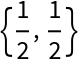Show it:

 In:=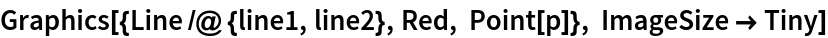Out=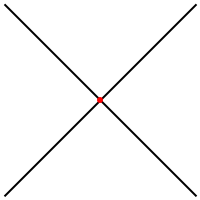Line may be used:

 In:=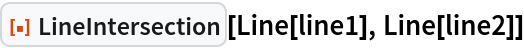Out=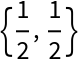A list of two lines may be used:

 In:=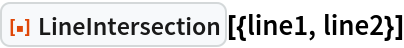Out=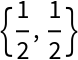### Scope (4)

Find the intersection of two infinite lines:

 In:=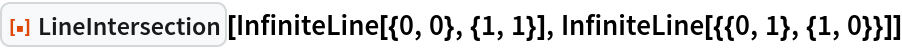Out=Two lines in 3D:

 In:=Find their intersection:

 In:=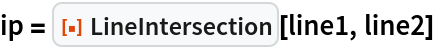Out=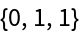Show the lines and the intersection point:

 In:=Out=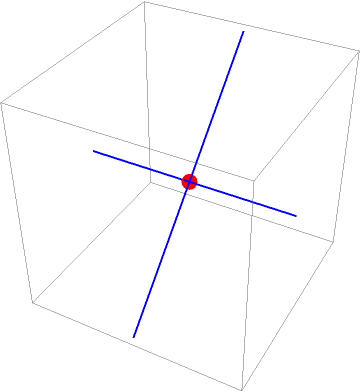Ten thousand line pairs:

 In:=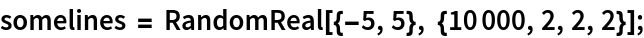Find the intersections and get timing:

 In:=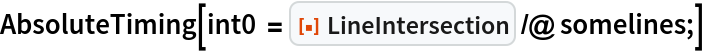Out=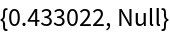In the documentation for InfiniteLine, the following method is used:

 In:=That method is slower:

 In:=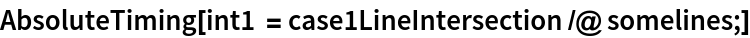Out=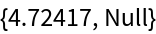RegionIntersection may also be used:

 In:=That method is also slower:

 In:=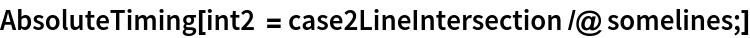Out=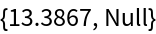All three methods give the same results:

 In:=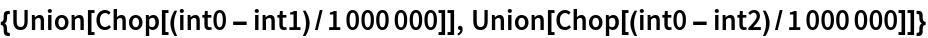Out=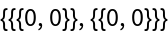Inexact values may be used:

 In:=Out=### Possible Issues (3)

The lines are considered to be infinite lines, thus the intersection point may not be on the defining segments:

 In:=Out=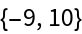Show it:

 In:=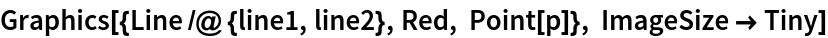Out=Degenerate lines will not return an intersection point:

 In:=Out=Parallel lines will not return an intersection point:

 In:=Out=## Version History

• 1.0.0 – 14 June 2022